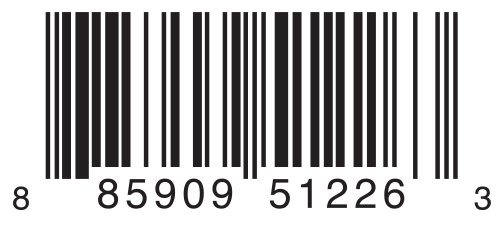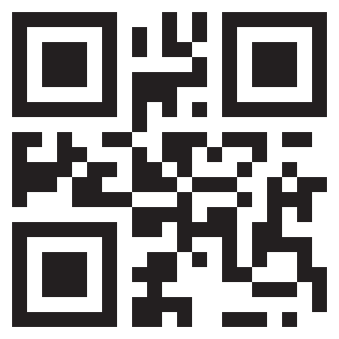# Swift学习之路-枚举

Swift 中的枚举是具有自己权限的一类类型。它们使用了许多一般只被类所支持的特性，例如计算属性用来提供关于枚举当前值的额外信息，并且实例方法用来提供与枚举表示的值相关的功能。枚举同样也能够定义初始化器来初始化成员值；而且能够遵循协议来提供标准功能。

## 枚举语法

``````enum SomeEnumeration {
// enumeration definition goes here
}``````

``````enum CompassPoint {
case north
case south
case east
case west
}``````

``````enum Planet {
case mercury, venus, earth, mars, jupiter, saturn, uranus, neptune
}``````

``var directionToHead = CompassPoint.west``

``directionToHead = .east``

## 使用 Switch 语句来匹配枚举值

``````directionToHead = .south
case .north:
print("Lots of planets have a north")
case .south:
print("Watch out for penguins")
case .east:
print("Where the sun rises")
case .west:
print("Where the skies are blue")
}
// prints "Watch out for penguins"``````

“判断 directionToHead的值。在等于 .north的 case中，则打印 "Lots of planets have a north"。在等于 .south的 case中，则显示 "Watch out for penguins"”

……以此类推。

``````let somePlanet = Planet.earth
switch somePlanet {
case .earth:
print("Mostly harmless")
default:
print("Not a safe place for humans")
}
// Prints "Mostly harmless"``````

## 遍历枚举情况（case）

``````enum Beverage: CaseIterable {
case coffee, tea, juice
}
let numberOfChoices = Beverage.allCases.count
print("\(numberOfChoices) beverages available")
// Prints "3 beverages available"``````

``````for beverage in Beverage.allCases {
print(beverage)
}
// coffee
// tea
// juice``````

## 关联值``````enum Barcode {
case upc(Int, Int, Int, Int)
case qrCode(String)
}``````

“定义一个叫做 Barcode的枚举类型，它要么用 `(Int, Int, Int, Int)`类型的关联值获取 upc 值，要么用 String 类型的关联值获取一个 qrCode的值。”

``var productBarcode = Barcode.upc(8, 85909, 51226, 3)``

``productBarcode = .qrCode("ABCDEFGHIJKLMNOP")``

``````switch productBarcode {
case .upc(let numberSystem, let manufacturer, let product, let check):
print("UPC: \(numberSystem), \(manufacturer), \(product), \(check).")
case .qrCode(let productCode):
print("QR code: \(productCode).")
}
// Prints "QR code: ABCDEFGHIJKLMNOP."``````

``````switch productBarcode {
case let .upc(numberSystem, manufacturer, product, check):
print("UPC : \(numberSystem), \(manufacturer), \(product), \(check).")
case let .qrCode(productCode):
print("QR code: \(productCode).")
}
// Prints "QR code: ABCDEFGHIJKLMNOP."``````

## 原始值

``````enum ASCIIControlCharacter: Character {
case tab = "\t"
case lineFeed = "\n"
case carriageReturn = "\r"
}``````

### 隐式指定的原始值

``````enum Planet: Int {
case mercury = 1, venus, earth, mars, jupiter, saturn, uranus, neptune
}``````

``````enum CompassPoint: String {
case north, south, east, west
}``````

``````let earthsOrder = Planet.earth.rawValue
// earthsOrder is 3

let sunsetDirection = CompassPoint.west.rawValue
// sunsetDirection is "west"``````

### 从原始值初始化

``````let possiblePlanet = Planet(rawValue: 7)
// possiblePlanet is of type Planet? and equals Planet.Uranus``````

``````let positionToFind = 11
if let somePlanet = Planet(rawValue: positionToFind) {
switch somePlanet {
case .earth:
print("Mostly harmless")
default:
print("Not a safe place for humans")
}
} else {
print("There isn't a planet at position \(positionToFind)")
}
// Prints "There isn't a planet at position 11"``````

## 递归枚举

``````enum ArithmeticExpression {
case number(Int)
indirect case multiplication(ArithmeticExpression, ArithmeticExpression)
}``````

``````indirect enum ArithmeticExpression {
case number(Int)
case multiplication(ArithmeticExpression, ArithmeticExpression)
}``````

``````let five = ArithmeticExpression.number(5)
let four = ArithmeticExpression.number(4)
let product = ArithmeticExpression.multiplication(sum, ArithmeticExpression.number(2))``````

``````func evaluate(_ expression: ArithmeticExpression) -> Int {
switch expression {
case let .number(value):
return value
return evaluate(left) + evaluate(right)
case let .multiplication(left, right):
return evaluate(left) * evaluate(right)
}
}

print(evaluate(product))
// Prints "18"``````